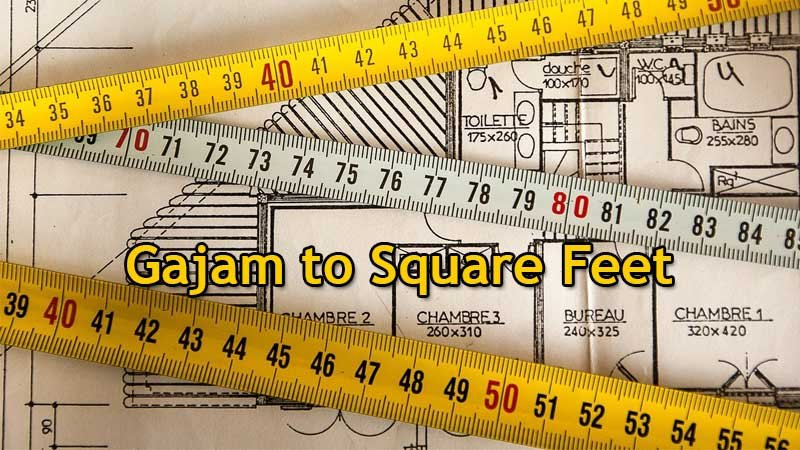# Gajam To Square Feet(Sq Ft) Converter

gajam means - a unit of land area measurement. This tool provides great calculation over gajam to sq ft, 1 gajam to sq ft, 1 gajam in feet, gajam to feet, 1 gajam equal to, 1 gajam in square feet and etc. To convert gajam to square feet, then multiply the unit value by 9. Then, 1 gajam * 9 = 9 Sq Ft. Hence, 1 gajam is equal to 9 Sq Ft.

Input Here

gajam

Output

sq ft

1 Gajam = 9 Square Feet
(1 Gajam = 9 Sq Ft)

Formula For Gajam to Square Conversion(gajam to sq ft formula)

The base formula for this gajam to sq ft converter is

Square Feet = Gajam * 9

How many square feet in a gajam?

Step 1: To Convert 1 gajam to sq ft

Step 2: Applying formula sq ft = gajam * 9, (i.e) multiply the unit value by 9.

Step 3: Then, sq ft = 1 * 9 = 9.

Step 4: Hence, 1 gajam is equal to 9 square feet.Some gajam to square feet Conversion Chart for your reference:

 1 gajam = 9 Square Feet 2 gajam = 18 Square Feet 3 gajam = 27 Square Feet 4 gajam = 36 Square Feet 5 gajam = 45 Square Feet 6 gajam = 54 Square Feet 7 gajam = 63 Square Feet 8 gajam = 72 Square Feet 9 gajam = 81 Square Feet 10 gajam = 90 Square Feet

The gajam to sq ft conversion chart is above listed for your reference. This chart, however, represents the simple math calculation involved in the gajam to square feet convertion online.

For Example: How much is 1 gajam to sq ft

Solution:

= (gajam * 9)

= (1 x 9)

= 9 Square Feet

For Example: How much is 120 gajam in square feet

Solution:

= (gajam * 9)

= (120 x 9)

= 1080 square feet

This formula provides an instant answer for all your questions / People also search:

• 1 gajam to square feet
• 1 gajam is equal to how many square feet
• Formula to convert gajam to sq ft online
• How to convert gajam to square feet?
• How much sq ft is equal to a one gajam?
• How many sq ft in gajam?
• 1 gajam equal to square feet